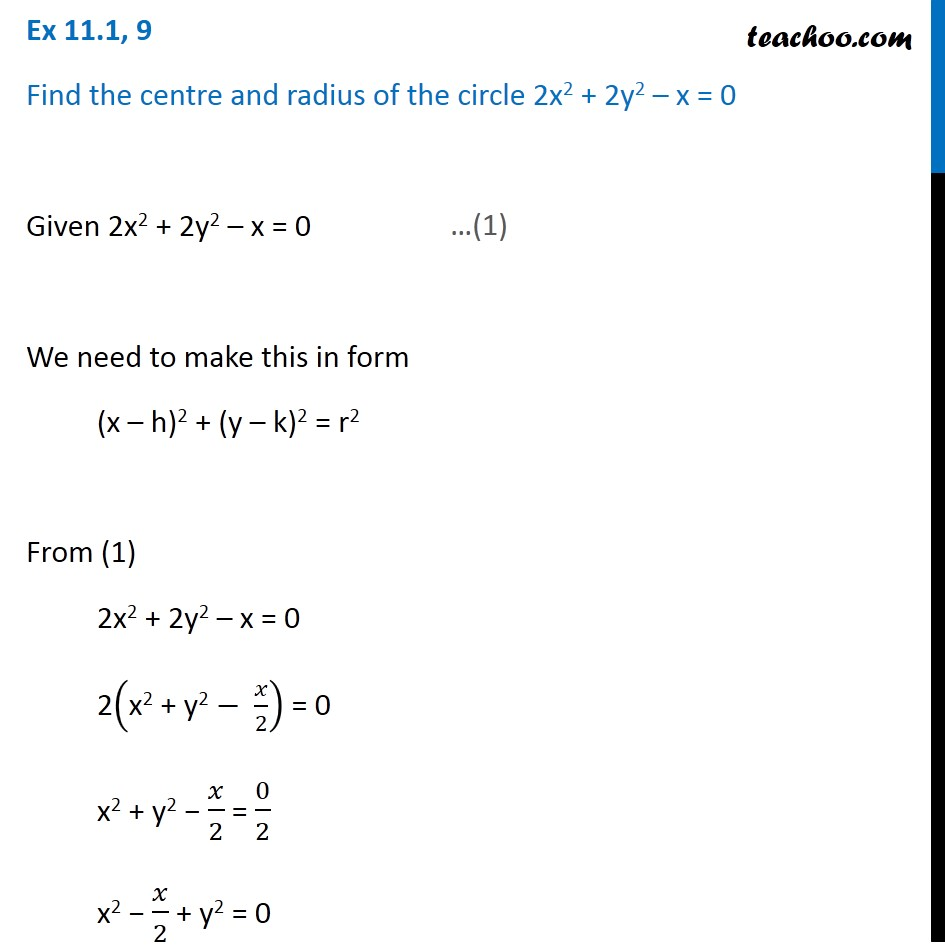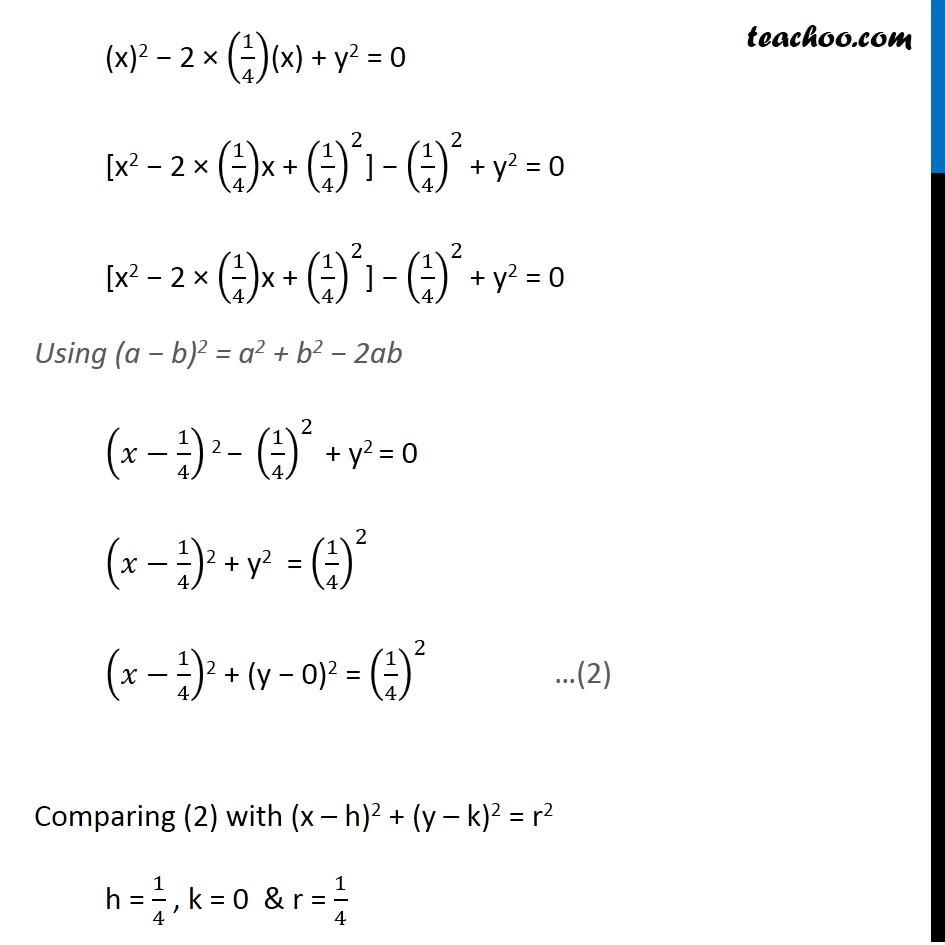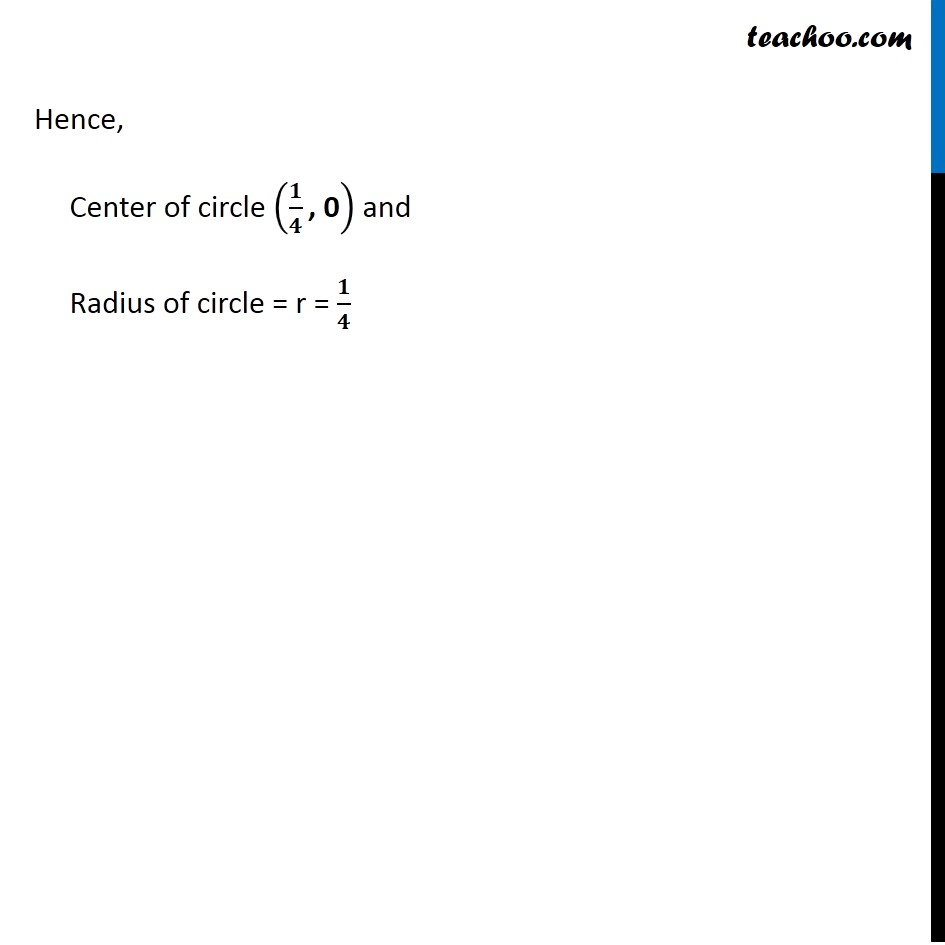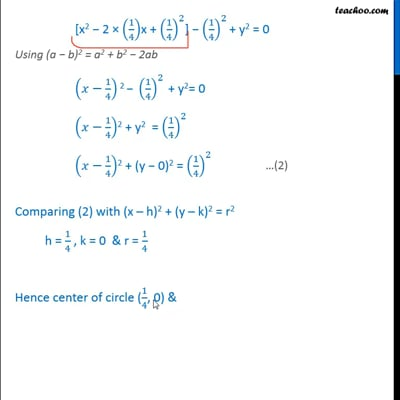Circle

Chapter 11 Class 11 Conic Sections
Concept wiseThis video is only available for Teachoo black users

Introducing your new favourite teacher - Teachoo Black, at only ₹83 per month

### Transcript

Ex 11.1, 9 Find the centre and radius of the circle 2x2 + 2y2 – x = 0 Given 2x2 + 2y2 – x = 0 We need to make this in form (x – h)2 + (y – k)2 = r2 From (1) 2x2 + 2y2 – x = 0 2("x2 + y2" − 𝑥/2) = 0 x2 + y2 − 𝑥/2 = 0/2 x2 − 𝑥/2 + y2 = 0 Ex 11.1, 9 Find the centre and radius of the circle 2x2 + 2y2 – x = 0 Given 2x2 + 2y2 – x = 0 We need to make this in form (x – h)2 + (y – k)2 = r2 From (1) 2x2 + 2y2 – x = 0 2("x2 + y2" − 𝑥/2) = 0 x2 + y2 − 𝑥/2 = 0/2 x2 − 𝑥/2 + y2 = 0 (x)2 − 2 × (1/4)(x) + y2 = 0 [x2 − 2 × (1/4)x + (1/4)^2] − (1/4)^2 + y2 = 0 [x2 − 2 × (1/4)x + (1/4)^2] − (1/4)^2 + y2 = 0 Using (a − b)2 = a2 + b2 − 2ab (𝑥−1/4)"2 − " (1/4)^2 " + y2 = 0" (𝑥−1/4)2 + y2 = (1/4)^2 (𝑥−1/4)2 + (y − 0)2 = (1/4)^2 Comparing (2) with (x – h)2 + (y – k)2 = r2 h = 1/4 , k = 0 & r = 1/4 Hence, Center of circle (𝟏/𝟒 ", 0" ) and Radius of circle = r = 𝟏/𝟒